• 在使用numpy函数库先要
千次阅读
2014-05-20 21:00:40

该系列文章是依据本人平时对机器学习的学习，归纳总结，所做的学习笔记。如有错误或待改善之处，请留下您宝贵的意见或建议。

# 1. NumPy函数库

NumPy函数库是Python的一种开源的数字扩展。这种工具可用来存储和处理大型矩阵，比Python自身的嵌套列表（nested list structure）结构要高效的多（该结构也可以用来表示矩阵（matrix））。

有关NumPy的相关资源可以访问官网：http://www.numpy.org/

机器学习算法涉及很多线性代数的知识，在用Python编写机器学习算法是会经常使用NumPy函数库。

首先，在确保可以正确运行Python开发环境的前提下，需要正确安装NumPy函数库。这里简单介绍在Ubuntu下安装NumPy库：

通过执行命令：sudo apt-get install python-numpy   和   sudo apt-get install python-scipy

在Python shell开发环境中输入下列命令：

>>> from numpy import *

上述命令将NumPy函数库中的所有模块引入到当前命名空间。接下来就可以在该空间中使用NumPy函数库了。

# 2. NumPy中矩阵与数组的区别

NumPy函数库中存在两种不同的数据类型（矩阵matrix和数组array），都可以用于处理行列表示的数字元素。虽然看起来很相似，但是这两个数据类型上执行相同的数学运算可能得到不同的结果，其中NumPy函数库中的matrix与MATLAB中的matrices等价。

该系列文章主要参考书目：<Machine Learning in Action>ubuntu
更多相关内容
• 1NumPy函数库是Python开发环境的一一个独立模块，而且大多数Python发行版没有默认安装NumPy函数库，因此安装Python之后必须单独安装NumPy函数库。2(1)Windows命令行提示符下输人c: \Python？\python. exe(2)通过...

1

NumPy函数库是Python开发环境的一一个独立模块，而且大多数Python发行版没有默认安装NumPy函数库，因此在安装Python之后必须单独安装NumPy函数库。

2

(1)Windows命令行提示符下输人c: \Python？\python. exe

(2)通过anaconda/anaconda Prompt进入

(3)Linux或者Mac OS的终端上输入python,进入Python sell开发环境。

今后，一旦看到“>>>”提示符就意味着我们已经进人Python sell开发环境: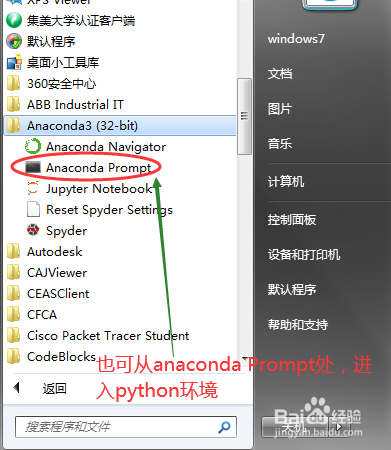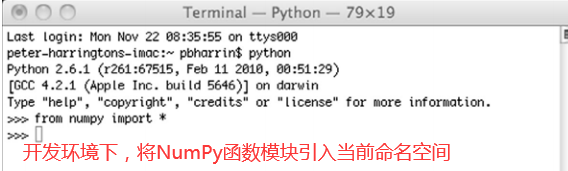3

在python开发环境中输入如下命令：

>>>import numpy as np

>>>np.random.rand(4,4)

此命令创造了一个4X4的随机数组,因为产生的是随机数组,不同计算机的输出结果可能与上述结果完全不同。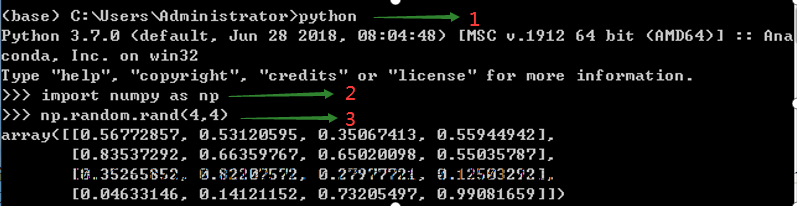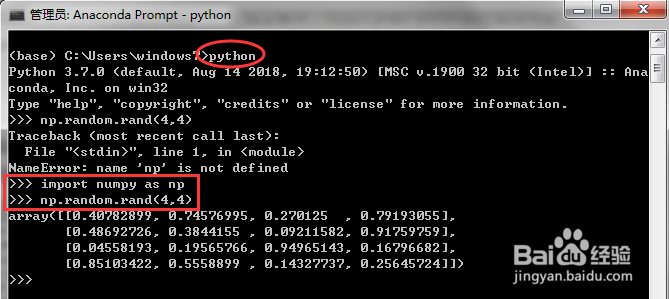4

调用mat()函数可以将数组转化为矩阵，输入如下命令：

>>>randMat=np.mat(np.romdom.rand(4,4))

>>>randMat.I # I实现了矩阵求逆的运算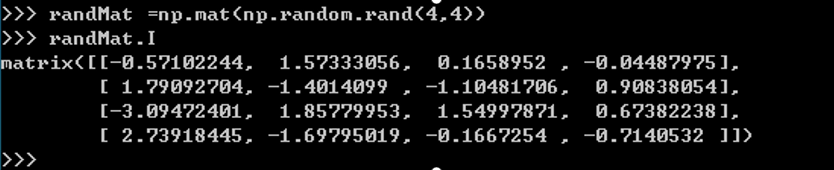5

(1)执行下面的命令存储逆矩阵：

>>>invRandMat = randMat.I

(2)接着执行矩阵乘法，得到矩阵与其逆矩阵相乘的结果：

>>>randMat*invRandMat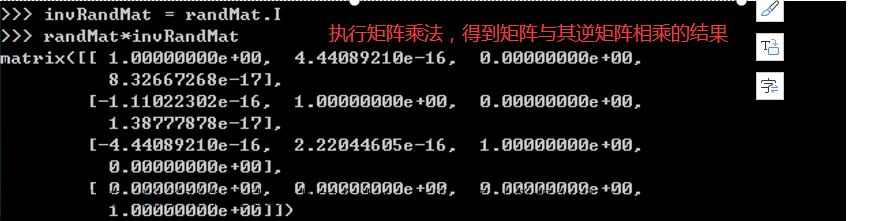6

输入下述命令，得到误差值：

>>>myEye= randMat*invRandMat>>>myEye - np.eye(4)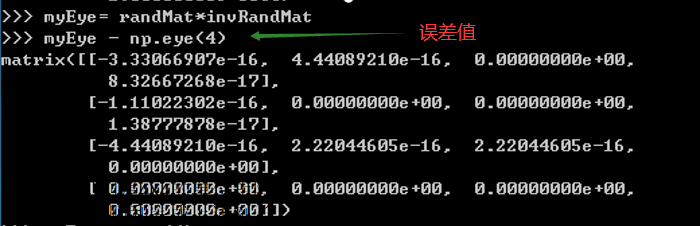7

函数eye(4)创建4X4的单位矩阵。

只要能顺利完成上述例子，就说明已经正确安装了NumPy函数库

END

展开全文• 机器学习算法涉及很多线性代数知识在使用Python语言构造机器学习应用时，会经常使用NumPy函数库。这里用到线性代数是为了简化不同的数据点上执行的相同数学运算。将数据表示为矩阵形式，只需要执行简单的矩阵运算而...

机器学习算法涉及很多线性代数知识在使用Python语言构造机器学习应用时，会经常使用NumPy函数库。这里用到线性代数是为了简化不同的数据点上执行的相同数学运算。将数据表示为矩阵形式，只需要执行简单的矩阵运算而不需要复杂的循环操作。在你开始学习机器学习算法之前，必须确保可以正确运行Python开发环境，同时正确安装了NumPy函数库。NumPy函数库是Python开发环境的一个独立模块，而且大多数Python发行版没有默认安装NumPy函数库，因此在安装Python之后必须单独安装 NumPy函数库。在Windows系统下安装numpy请参考https://blog.csdn.net/qq_43354004/article/details/105162767。
（一）构造随机数组
先输入from numpy import * 命令将NumPy函数库中的所有模块引入当前的命名空间。接着开始构造一个4x4的随机数组，因为产生的是随机数组，不同计算机的输出结果可能与上述结果完全不同。示例如下：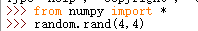执行结果：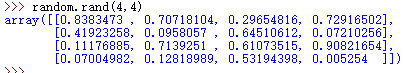（二）调用mat()函数将数组转化为矩阵
NumPy函数库中存在两种不同的数据类型（矩阵matrix和数组 array），都可以用于处理行列表示的数字元素。虽然它们看起来很相似，但是在这两个数据类型上执行相同的数学运算可能得到不同的结果，其中NumPy函数库中的matrix与MATLAB中matrices等价。
调用mat()函数可以将数组转化为矩阵，在后面加上.I操作符可以实现矩阵求逆的运算。示例如下：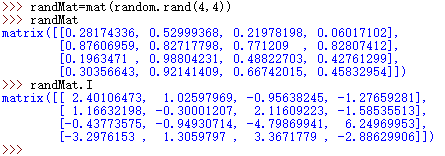（三）矩阵的乘法
使用*执行矩阵乘法，得到矩阵与其逆矩阵相乘的结果。示例如下：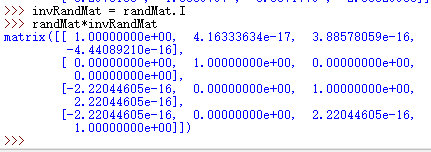结果应该是单位矩阵，除了对角线元素是1，4x4矩阵的其他元素应该全是0。实际输出结果略有不同，矩阵里还留下了许多非常小的元素， 这是计算机处理误差产生的结果。输入下述命令，得到误差值。函数eye(4)创建4×4的单位矩阵。示例如下：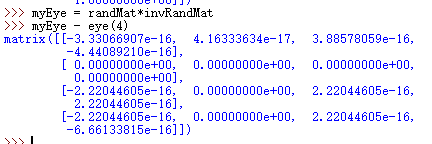展开全文python 机器学习
• numpy函数库中的所有模块引入：from numpy import *3. 构造一个4*4的随机数组：randArray = random.rand(4,4) print(randArray)4.mat()函数：将数组转化为矩阵：randMat = mat(random.rand(4,4)) print(randMat)5...

1. 安装numpy库：pip install  numpy

2. 将numpy函数库中的所有模块引入：

from numpy import *

3. 构造一个4*4的随机数组：

randArray = random.rand(4,4)
print(randArray)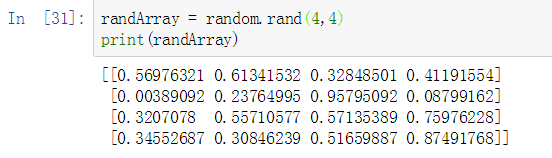4.mat()函数：将数组转化为矩阵：

randMat = mat(random.rand(4,4))
print(randMat)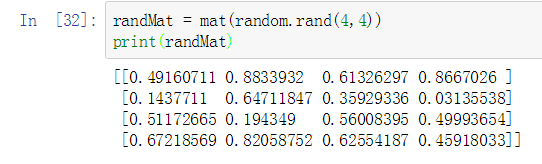5. 求逆矩阵.I操作符

randMat = mat(random.rand(4,4))
print(randMat)
invRandMat = randMat.I
print(invRandMat)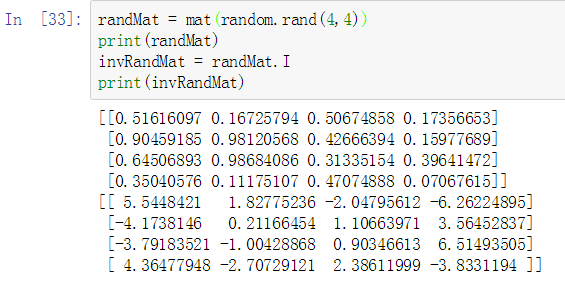6.执行矩阵乘法：

randMat = mat(random.rand(4,4))
print(randMat)
invRandMat = randMat.I
print(invRandMat)
Multi = randMat*invRandMat
print(Multi)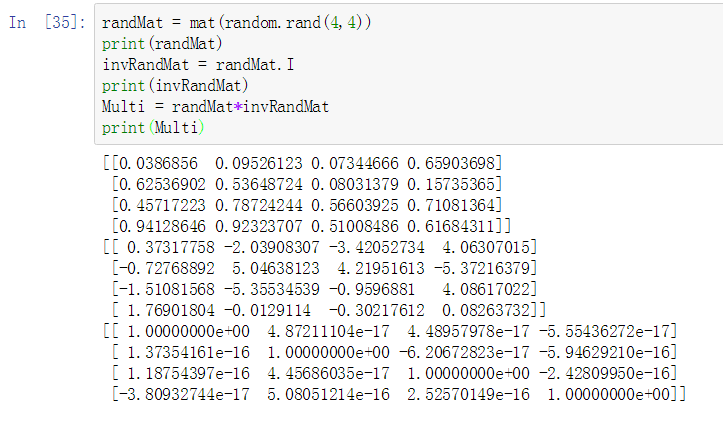结果应收是单位矩阵，除了对角线元素为1，其他元素应该为0，实际的输出略有不同，因为矩阵例还留下了许多非常小的元素，这是计算机处理误差产生的结果。

import numpy
vector = numpy.array([5,10,15,20])
matrix = numpy.array([[5,10,15],[20,25,30]])
print(vector)
print(matrix)
print(vector.shape)
print(matrix.shape)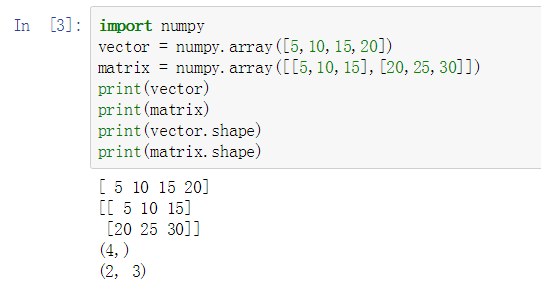.dtype查看类型

import numpy
numbers = numpy.array([1,2,3,4])
print(numbers)
numbers.dtype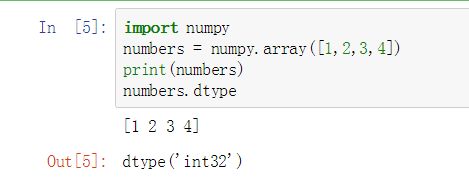import numpy
numbers = numpy.array([1,2,3,4.0])
print(numbers)
numbers.dtype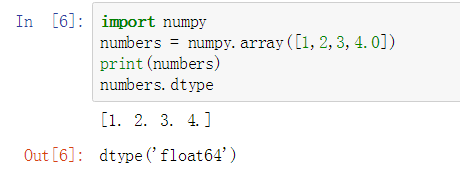import numpy
vector = numpy.array([5,10,15,20])
vector == 10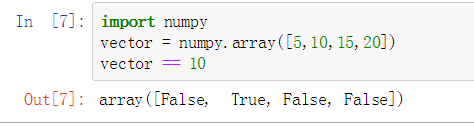import numpy
matrix = numpy.array([
[5,10,15],
[20,25,30],
[35,40,45]
])
matrix == 25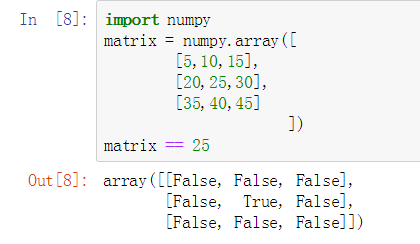import numpy
vector = numpy.array([5,10,15,20])
equal_to_ten = (vector == 10)
print (equal_to_ten)
print(vector[equal_to_ten])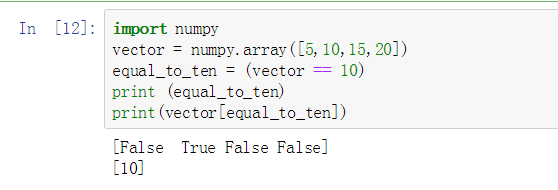与运算和或运算：

import numpy
vector = numpy.array([5,10,15,20])
equal_to_ten_and_five = (vector == 10) & (vector == 5)
print (equal_to_ten_and_five)import numpy
vector = numpy.array([5,10,15,20])
equal_to_ten_or_five = (vector == 10) | (vector == 5)
print (equal_to_ten_and_five)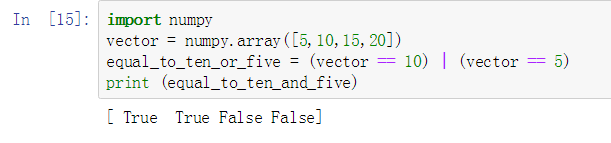类型转换：

import numpy
vector = numpy.array(["5","10","15","20"])
print(vector.dtype)
print(vector)

vector = vector.astype(float)
print(vector.dtype)
print(vector)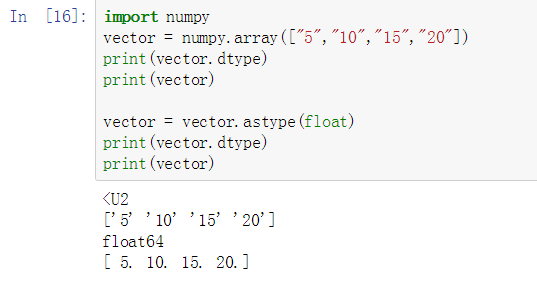求最小、最大值：

import numpy
vector = numpy.array([5,10,15,20])
min = vector.min()
print(min)
max = vector.max()
print(max)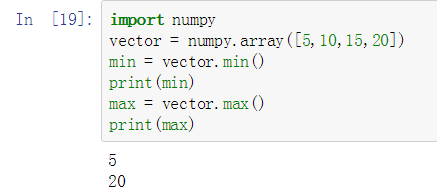计算每一行的和值：

import numpy
matrix = numpy.array([
[5,10,15],
[20,25,30],
[35,40,45]
])
matrix.sum(axis=1)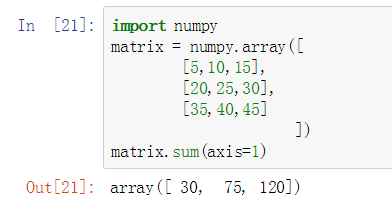计算每一列的和值：

import numpy
matrix = numpy.array([
[5,10,15],
[20,25,30],
[35,40,45]
])
matrix.sum(axis=0)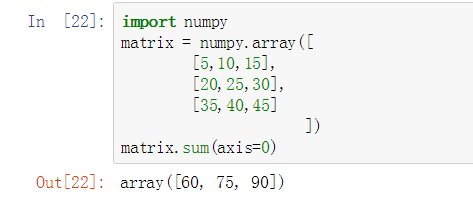import numpy as np #用np代替numpy
print(np.arange(15))
a = np.arange(15).reshape(3,5)
print(a)
a.shape
a.size #当前矩阵包含多少个元素
a.ndim  #当前矩阵的维度
a.dtype.name #当前矩阵的类型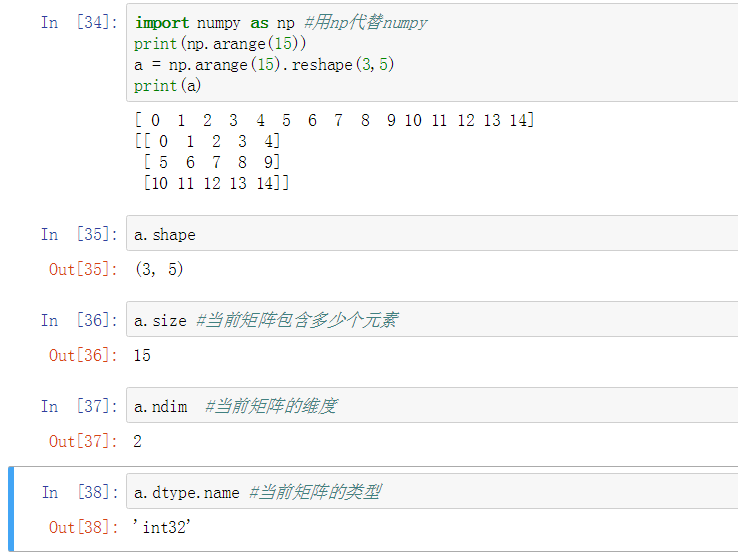import numpy
vector = numpy.array([5,10,15,20])
matrix = numpy.array([[5,10,15],[20,25,30]])
print(vector)
print(matrix)
print(vector.shape)
print(matrix.shape).dtype查看类型

import numpy
numbers = numpy.array([1,2,3,4])
print(numbers)
numbers.dtypeimport numpy
numbers = numpy.array([1,2,3,4.0])
print(numbers)
numbers.dtypeimport numpy
vector = numpy.array([5,10,15,20])
vector == 10import numpy
matrix = numpy.array([
[5,10,15],
[20,25,30],
[35,40,45]
])
matrix == 25import numpy
vector = numpy.array([5,10,15,20])
equal_to_ten = (vector == 10)
print (equal_to_ten)
print(vector[equal_to_ten])与运算和或运算：

import numpy
vector = numpy.array([5,10,15,20])
equal_to_ten_and_five = (vector == 10) & (vector == 5)
print (equal_to_ten_and_five)import numpy
vector = numpy.array([5,10,15,20])
equal_to_ten_or_five = (vector == 10) | (vector == 5)
print (equal_to_ten_and_five)类型转换：

import numpy
vector = numpy.array(["5","10","15","20"])
print(vector.dtype)
print(vector)

vector = vector.astype(float)
print(vector.dtype)
print(vector)求最小、最大值：

import numpy
vector = numpy.array([5,10,15,20])
min = vector.min()
print(min)
max = vector.max()
print(max)计算每一行的和值：

import numpy
matrix = numpy.array([
[5,10,15],
[20,25,30],
[35,40,45]
])
matrix.sum(axis=1)计算每一列的和值：

import numpy
matrix = numpy.array([
[5,10,15],
[20,25,30],
[35,40,45]
])
matrix.sum(axis=0)import numpy as np #用np代替numpy
print(np.arange(15))
a = np.arange(15).reshape(3,5)
print(a)
a.shape
a.size #当前矩阵包含多少个元素
a.ndim  #当前矩阵的维度
a.dtype.name #当前矩阵的类型展开全文Python
• 本文档为开源的numpy函数库，详细介绍了各种函数的使用方式，方便找不到资源的用户下载。
• 1、NumPy函数库是Python开发环境的一个独立模块，大多数Python发行版没有默认安装NumPy函数库，因此安装Python后必须单独安装NumPy函数库 2、http://sourceforge.net/projects/numpy/files/下载地址 3、Python...
• 1首先下载numpy函数库，下载链接https://www.lfd.uci.edu/~gohlke/pythonlibs/#numpy 。注意选择与自己Python版本匹配的numpy。我是Python2.7版本，windows64位，所以我下载的是numpy-1.16.5+mkl-cp27-cp27m-win_amd...
• 没给大家介绍numpy之前给大家说下python的基本概念。 Python 是一种高级的，动态的，多泛型的编程语言。Python代码很多时候看起来就像是伪代码一样，因此你可以使用很少的几行可读性很高的代码来实现一个非常...
• 1.安装pip 去网站https://raw.github.com/pypa/pip/master/contrib/get-pip.py 进入页面，右键另存为，将源码保存到本地，然后执行 get-pip.py 命令。编辑环境变量 ...该网站 https://www.lfd.uci....
• NumPy函数库   NumPy函数库是机器学习的基础库，也是我最近学习机器学习时发现的一个基础函数库。NumPy是Python语言的一个扩充程序库。支持高级大量的维度数组与矩阵运算，此外也针对数组运算提供大量的数学函数库...python 机器学习
• 转载于:https://www.cnblogs.com/ltxblog/p/8709079.html
• Python+opencv+numpy函数库！用于开发照片处理的
• 如果安装numpy函数库遇到问题建议用清华镜像安装 __________________________________________________________ 本次测试图： 效果图： 因为这次用到numpy库和image库，由于这二个库的知识点有点多，我就不做介绍
• NumPy函数库是Python开发环境的一个独立模块，而且大多数Python发行版没有默认安装NumPy数据库，因此安装Python之后必须单独安装NumPy数据库。 进入Python shell开发环境后输入 from numpy import* 如果出现No ...Python
• 1. NumPy安装 使用pip包管理工具进行安装 复制代码 代码如下: $sudo pip install numpy 使用pip包管理工具安装ipython(交互式shell工具) 复制代码 代码如下:$ sudo pip instlal ipython \$ ipython –pylab #pylab...
• 1.从官网下载Windows，x86版本python安装文件（安装选项勾选pip安装工具），地址：...cmd下进入Python的Scripts目录，键入命令 pip install wheel 可以通过pip freeze --all命令来判断是否正确安装Python
• 1. mean() 函数定义： numpy.mean(a, axis=None, dtype=None, out=None, keepdims=)[source] Compute the arithmetic mean along the specified axis. Returns the average of the array elements. The average ...
• NumPy(Numerical Python) 是 Python 语言的一个扩展程序库，支持大量的维度数组与矩阵运算，此外也针对数组运算提供大量的数学函数库NumPy 是一个运行速度非常快的数学库，主要用于数组计算，包含： 一个强大的...python 数据挖掘
• 支持多维数组与矩阵运算，此外也针对数组运算提供大量的数学函数库Numpy是人工智能、数据分析从业者必备的知识和技能，也是学习后续Python扩展库（Matplotlib, SciPy, Pandas, Seaborn, Scikit-image等）的基础。 ...人工智能 机器学习 python
• ## Numpy常用函数汇总

千次阅读 2022-01-28 22:48:56
文章目录1 创建数组1.1 使用array（）导入向量1.2 numpy.array()也可以用来导入矩阵2 创建Numpy数组2.1 创建全0矩阵2.2 创建多维矩阵2.3 创建全1矩阵2.4 创建一个用指定值填满的矩阵2.5 生成一个指定范围的矩阵2.6...python
• 模块8：numpy库使用   要点：numpy是用于处理含有同种元素的多维数组运算的第三方。 1.numpy库概述 Python标准中提供了一个array类型，用于保存数组类型数据，然而这个类 型不支持多维数据，处理函数也不够...python
• 个人学习机器学习时总结的numpy函数，包含整个《机器学习实战》，《机器学习》，《python编程：从入门到实践》的所有numpy函数的功能与用法。绝对实用，内部含有详细的代码解释
•python
• Python之Numpy库常用函数大全（含注释）
• NumPy(Numerical Python) 是 Python 语言的一个扩展程序库，支持大量的维度数组与矩阵运算，此外也针对数组运算提供大量的数学函数库想安装NumPy有两种方式，第一种是 cmd 中安装；第二种是 pycharm...
• ## Numpy库的安装

千次阅读 2021-11-19 10:28:12
NumPy提供了一个非常好的，用于简单(编写代码方面)和快速(速度方面)计算。NumPy数组用于存储训练数据和机器学习模型的参数。 **图像处理和计算机图形学：**计算机中的图像表示为多维数字数python 机器学习 计算机视觉
• ## NumPy函数

千次阅读 2021-01-15 02:01:18...